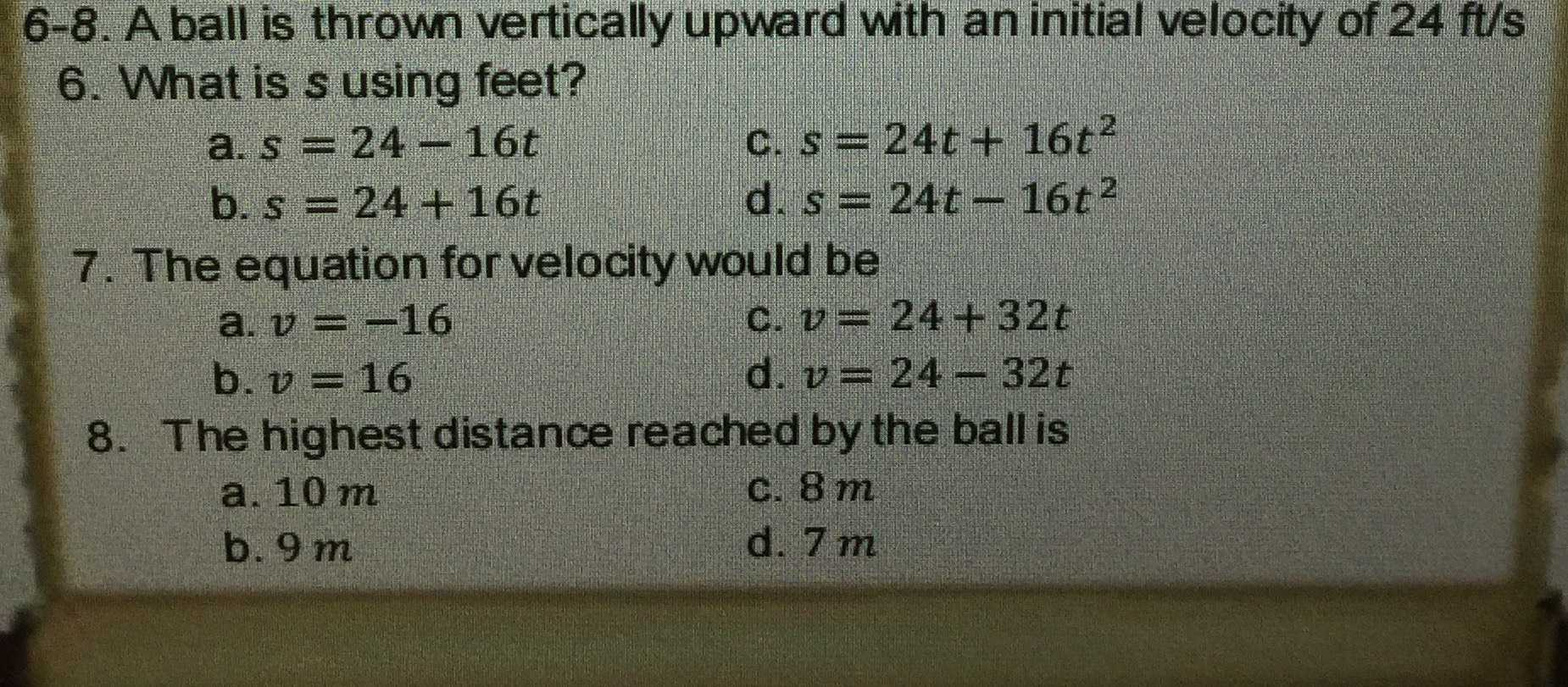### ¿Todavía tienes preguntas de matemáticas?

Pregunte a nuestros tutores expertos
Algebra
PreguntaA ball is thrown vertically upward with an initial velocity of $$24 ft / s$$

6. What is $$s$$ using feet?

a. $$s = 24 - 16 t$$ c. $$s = 24 t + 16 t ^ { 2 }$$

b. $$s = 24 + 16 t$$ d. $$s = 24 t - 16 t ^ { 2 }$$

7. The equation for velocity would be

a. $$v = - 16$$ c. $$v = 24 + 32 t$$

b. $$v = 16$$     d. $$v = 24 - 32 t$$

8. The highest distance reached by the ball is

a. $$10 m$$ c. $$8 m$$

b. $$9 m$$    d. $$7 m$$